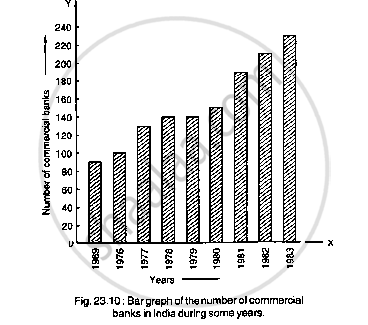# Read the Bar Graph Shown in Fig. 23.10 and Answer the Following Questions (I) What is the Information Given by the Bar Graph?(Ii) What Was the Number of Commercial Banks in 1977? - Mathematics

Read the bar graph shown in Fig. 23.10 and answer the following questions
(i) What is the information given by the bar graph?(ii) What was the number of commercial banks in 1977?
(iii) What is the ratio of the number of commercial banks in 1969 to that in 1980?
(iv) State whether true or false:
The number of commercial banks in 1983 is less than double the number of commercial banks in 1969.

#### Solution

(i) Given bar graph represents the number of commercial banks in India during some years.
(ii) The number of commercial banks in 1977 was

120+(140-120)/2

=120+20/2

=120+10

= 130.
(iii) The ratio of the number of commercial banks in 1969 was

80 +(100-80)/2

=80+20/2

=80+10

= 90

The number of commercial banks in 1980 was

140+(160-140)/2

=140+20/2

= 140+10

= 150

Hence, the required ratio is

90/150

=3/5

=3: 5
(iv) The number of commercial banks in 1983 was

220+(240-220)/2

220+20/2

=220+10
= 230

The number of commercial banks in 1969 was 90. When we multiply this number by 2, it becomes

2 × 90

= 180

Clearly, 230 is not less than 180.

Hence the statement is false.

Concept: Graphical Representation of Data
Is there an error in this question or solution?

#### APPEARS IN

RD Sharma Mathematics for Class 9
Chapter 23 Graphical Representation of Statistical Data
Exercise 23.1 | Q 6 | Page 9

Share